Next: Improving Alignments With Gap Up: Methods Previous: Methods

## An Algorithm For Finding All Possible Gap Blocks

For simplicity, in the following each gap is represented by an integer which is its length. All gaps of the MSA are represented by L:

Definition 3.1   The gaps of an MSA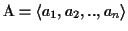are represented as an ordered list L of lists,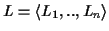, where each Li is the sequence of gaps in ai. |Li| is the number of gaps in ai, and the element Lij is the length of the jth gap in ai.

Definition 3.2   A gap block B of an MSAis defined as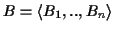, where each Bi is either a contiguous subsequence denoted by the first and last indices in Li (i.e. Bi[first], Bi[last]) or Bi is empty. For each non empty Bi, the sum of the gap lengths must be the same: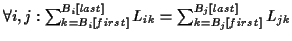.

All possible B's are considered except for:
a)
Whenever there are gaps in different sequences at the same position of the same length, we assume that they are placed correctly, because such gaps already are in a block, and we do not need to disrupt them (e.g. if L1,j1 = L2,j1 = L3,j1 and the three gaps start at the same place in the MSA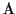, we treat them as one gap).
b)
If the gaps start more than W characters away from each other in the MSA, then we discard them.
c)
We only consider rmax gaps at a time:Problem 3.1 (Find all gap blocks)   Given is an MSA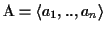. The problem is to find all possible gap blocks B in(see Definition).

Example 3.1:  The MSA in Figureis transformed into a list. From this list, many possible gap blocks can be formed. Two valid gap blocks are shown on the left in Figure(empty lists are not shown). In both, B1 and B2, each B1i, B2i is a subsequence of Li, and the sum of the integers in each B1i, B2i is the same. One invalid gap block is shown on the right in Figure. In B3, the sum of the lengths in one B3i is not the same.

Figure: Each sequence of the MSA is transformed to a list L of integers

 a1 PDCVCPTLKQAAKAV____RLQG_QHQ____PMQ_______VRKIYQTAKHLPNVC a2 PVCVCPTLRQAARAV____SLQG_QHG____PFQ_______SRKIYKTAKYLPNIC a3 PVCVCPTLKQAARAV____SLQG_QHG____PFQ_______SRKIYQSAKYLPNIC a4 QVCVCPTLKQAAKSVRVQGQHG________________PFQSTRIYQIAKNLPNVC a5 PLCVCPTLKGASKAVKQQVRQQLEQQG____Q____QGPHVISRIYQTATHLPKVC a6 PLCVCPTLKGASKAVKQQVRQ___QQGQQGQQLQQV_____ISRIYQTATHLPKVC a7 PLCVCPTLKGASKAVRQQVRQ___QQG___QQMQGQQMQQVISRVYQTATHLPRVC a8 PLCVCPTLKGASKAVKQQIRQQGQQQGQQGQQLQHE_____ISRIYQTATHLPRVC a9 PLCVCPTLKGAAKAVKQQIQQQGQQHGQQGQQLQHE_____IRRIYQTATHLPKVC a10 PLCVCPTLKGASKAVKQQIQQQGQQQG__KLQM________VSRIYQTATHLPKVC a11 PLCVCPTLKGASKAVKQQIQQQGQQQG__KQQM________VSRIYQTATHLPKVC a12 PLCVCPTLRGASKAVKQQIQQQEQ__QQGKQQM________VNRIYQTATHLPKVC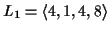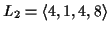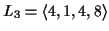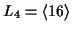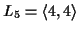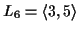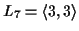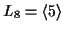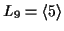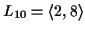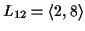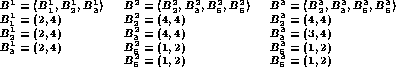Most proteins are composed of domains, which are usually at most 70 amino acids long. In order to find all possible gap blocks, we restrict the problem to the size of a domain, because it does not make sense biologically to combine gaps which are further from each other than a domain size. Usually we don't know where a domain starts and where it ends, therefore we define a window of width W and height n that slides along the MSA. W is the maximum number of characters in one sequence of the MSA we look at. Within this window, we want to find all combinations of gaps that build blocks. In the following, when we use L, we mean an L restricted by such a window.

Definition 3.3   The parameter W is the maximum number of positions we analyze at in a sequence of an MSA. Let wi be the maximum possible number of gaps that can appear in Li for a window of size W. It is clear that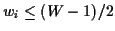and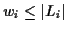.

In addition, we restrict the maximum number of gaps in each sequence we will combine to a block.

Definition 3.4   The parameter rmax is the maximum number of gaps that can be contained in any Bi of a gap block B.

Let us now determine the number of possible gap blocks that can be formed. To build a gap block B, for each row we can either choose gaps or not. Since there are at most w gaps in each row for each position, we can buildblocks in the worst case, or more precisely,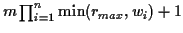, when m is the length of a sequence ai in the MSA.
Procedure 3.1: FindAllBlocks
Input: L, rmax
Output: a list of gap blocks P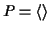;
for r = 1 to rmax do by 1em by -1em
for i = 1 to n do by 1em by -1em
compute the list P' of all possible blocks such that for each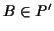: by 1em by -1em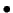Bi = (1, r) andfor all k > i,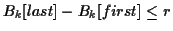and
Bk = (1, s) s.t.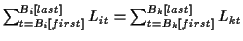;
for all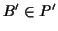do by 1em by -1em
if B' contains more than one gap then add B' to P;
L' = copy(L);
remove all elements from L' that correspond to B';
if L' is not empty then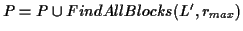; by -1em by 1em
od; by -1em by 1em
od; by -1em by 1em
od;
return P; by -1em by 1em
In real cases, the number of gaps is small enough so that the problem is solvable in a reasonable amount of time for r up to 5 and for W up to around 70 (see Section 3.3).Next: Improving Alignments With Gap Up: Methods Previous: Methods
Chantal Korostensky
1999-07-14